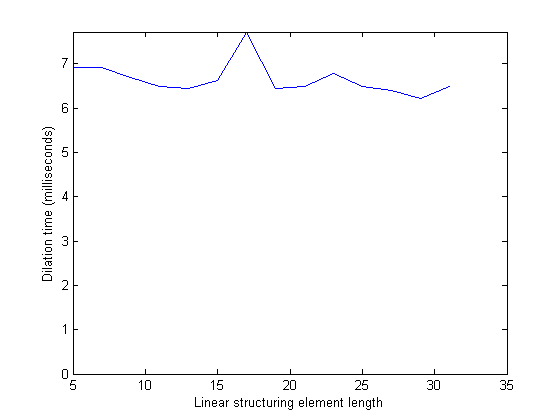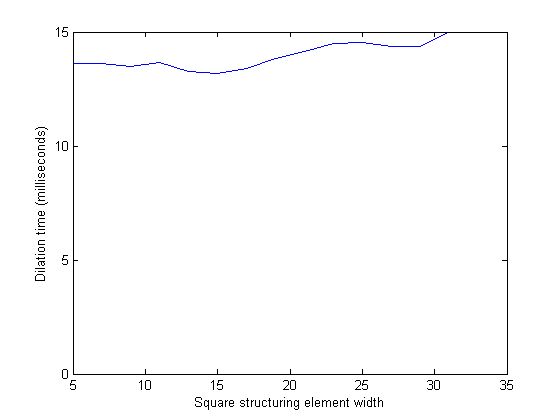# Dilation with linear structuring elements

For my series on dilation algorithms, I've followed my unfortunate custom of dragging a series out way too long. :-)

So let's wrap it up with a mention of the last key algorithmic idea implemented in the Image Processing Toolbox morphology functions: grayscale dilation and erosion with horizontal and vertical structuring elements.

Normally, the time required to perform dilation and erosion is proportional to the number of elements in the domain of the structuring element. But there are specialized algorithms that achieve better performance for certain classes of structuring elements. In particular, imdilate and imerode use the algorithm described in M. van Herk, "A fast algorithm for local minimum and maximum filters on rectangular and octagonal kernels," Pattern Recognition Letters, 13:517-521, 1992. There's a helpful description of this algorithm in P. Soille, Morphological Image Analysis: Principles and Applications, 2nd ed., 2007, 89-90.

This algorithm can perform flat grayscale dilation using horizontal and vertical linear structuring elements in time independent of the length of the strel.

Let's test that.

I = imread('rice.png');
lengths = 5:2:31;
times = zeros(size(lengths));
for k = 1:numel(lengths)
se = strel(ones(1, lengths(k)));
times(k) = timeit(@() imdilate(I, se));
end

plot(lengths, 1000*times)
ylim([0 max(1000*times)])
xlabel('Linear structuring element length')
ylabel('Dilation time (milliseconds)')For rectangular structuring elements, this algorithm interacts especially nicely with structuring element decomposition. The functions imdilate and imerode automatically detect flat, rectangular structuring elements and decompose them into horizontal and vertical line structuring elements. Therefore, grayscale dilation and erosion with flat, rectangular structuring elements can also be performed in time independent of the structuring element size.

Here's a test for square structuring elements.

lengths = 5:2:31;
times = zeros(size(lengths));
for k = 1:numel(lengths)
se = strel(ones(lengths(k), lengths(k)));
times(k) = timeit(@() imdilate(I, se));
end

plot(lengths, 1000*times)
ylim([0 max(1000*times)])
xlabel('Square structuring element width')
ylabel('Dilation time (milliseconds)')That's a nice result, especially since using rectangular structuring elements is fairly common.

To recap, the main dilation and erosion algorithm ideas exploited by Image Processing Toolbox morphology functions are:

• structuring element decomposition
• binary bit packing
• specialized algorithms for horizontal and linear strels

You can see all the posts in the series by clicking on the "Dilation algorithms" category link on the right side of the page.

OK, that's it - I'm signing off for 2008.

Happy New Year, everyone!

Published with MATLAB® 7.7

|